NEET  >  Industrial Applications of Le Chatelier's Principle & Haber Process

# Industrial Applications of Le Chatelier's Principle & Haber Process - Notes | Study Chemistry Class 11 - NEET

 1 Crore+ students have signed up on EduRev. Have you?

INDUSTRIAL APPLICATIONS OF LE CHATELIER'S PRINCIPLE

1. HABER PROCESS

In Haber process, the ammonia is synthesized by combining pure nitrogen and hydrogen gases in 1:3 ratio in presence of finely powdered iron catalyst and molybdenum promoter at around 450oC and at about 250 atm. of pressure.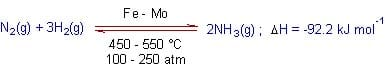The le Chatelier's principle helps in choosing these conditions to improve the yields of ammonia as explained as below.

Effect of pressure:

Hence the synthesis of ammonia is favored by increasing the pressure of the system. Industrially, 100 - 250 atm. of pressure is employed. Effect of temperature: Since the forward reaction is exothermic, the increase in temperature favors the backward reaction i.e., the dissociation of ammonia. That means according to le Chatelier's principle, the synthesis of ammonia is favored at lower temperatures. However the reaction will be too slow at lower temperatures (a kinetic restriction). Hence this reaction is carried out at optimal temperatures i.e., at about 450 - 550 oC to overcome the kinetic barrier. Removal of ammonia: The forward reaction can also be favored by removing ammonia from the system from time to time by liquefying it. Catalyst: To increase the speed of the reaction, finely powdered or porous iron is used as catalyst. Its efficiency can be improved by adding molybdenum or oxides of potassium and aluminium.

 The Percent by Mass of NH3 at Equilibrium in a Mixture of N2 ,H2 and NH3 as a Function of T and Total pressure Total Pressure Temperature (°C) 300 atm 400 atm 500 atm 400 48% NH3 55% NH3 61% NH3 500 26% NH3 32% NH3 38% NH3 600 13% NH, 17% NH, 21% NH,

2. CONTACT PROCESS

In the contact process, sulfuric acid, the king of chemicals, is manufactured on large scale. The major steps involved in the process are: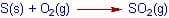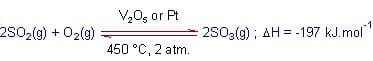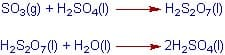The crucial step is the oxidation of sulfur dioxide, SO2 to sulfur trioxide, SO3. It is a reversible reaction. At normal conditions, the equilibrium lies far to the left and the amount of sulfur trioxide formed is very small. To improve the yield of sulfur trioxide, the reaction is carried out at around 450oC and 2 atm pressure in presence of V2O5 or Pt, which acts as a catalysts.

These conditions are chosen by applying le Chatelier's principle as explained below.

Effect of pressure: In the forward reaction (formation of sulfur trioxide), the number of moles of gaseous components is decreasing.

Hence the forward reaction is favored by increasing the pressure of the system. However, at high pressures, the iron towers used in the contact process are corroded. Hence the process is carried out at optimal pressures like 2 atm.

Effect of temperature: Since the forward reaction is exothermic, at higher temperatures the backward reaction i.e., the dissociation of sulfur dioxide is more favored. However the reaction will be too slow at lower temperatures. Hence this reaction is carried out at optimal temperatures i.e., around 450° C.

Catalyst: To increase the speed of the reaction, V2O5 or Pt are used as catalysts

Ex. Under what conditions will the following reactions go in the forward direction?

1. N2(g) + 3H2(g)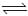2NH3(g) +23 Kcal.

2. 2SO2(g) + O2(g)2SO3(g)+ 45 Kcal.

3. N2(g) + O2(g)2NO(g) - 43.2 Kcal

4. 2NO(g) + O2(g)2NO2(g)+ 27.8 Kcal

5. C(s) + H2O(g)CO2(g) + H2(g) + x k cal.

6. PCl5(g)PCl3(g) + Cl2(g) - X kcal.

7. N2O4(g)2NO2(g) - 14 kcal

Sol. 1. Low T, High P, excess of N2 and H2 .

2. Low T, High P, excess of SO2 and O2

3. High T, any P , excess of N2 and O2

4. Low T, High P, excess of NO and O2

5. Low T, Low P, excess of C and H2O

6. High T, low P, excess of PCl5

7. High T, Low P, excess of N2O4

Simultaneous Equilibrium

A(s)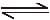B(g) + C(g)

D(s)B(g) + E(g)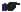Applicable only when at least one of the product is common in both the reaction.

Ex. The pressure at equilibrium over solid A is 50 atm and over solid D is 68 atm if both solid A and D are heated simultaneously then find

(i) the total pressure over the solids.

(ii) In the above question find the mole ratio of C and B

(iii) mole fraction of C

Sol. (i)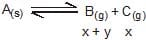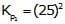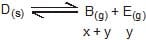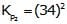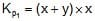...(i)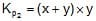...(ii)

total pressure = pB + pC + pE = x+ y+ x +y = 2 (x+ y)

Also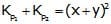→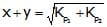→ Total pressure = 2 (x + y) =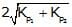(ii) At constant temp and volume P ≈ n

→ pressure Ratio will their mole ratio by eq. (i)/(ii)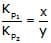(iii) Mole fraction of C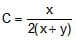As we know→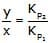→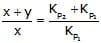,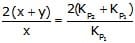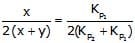→ mole fraction of C =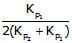Ex.6 A(s)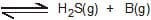At eq., pressure = 18 atm

C(s)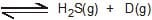At eq., pressure = 36 atm

Calculation

(i) total pressure at new equilibrium when both the solids are heated simultaneously.

(ii) mole ratio of B and D

(iii) mole fraction of B in the mixture.

Sol. A(s)H2S(g) + B(g), Kp1 = (9)= 81

x + y        x

C(s)H2S(g) + D(g), Kp2 = (18)2 = 324

x + y        y

total pressure = x + y + x + y = 2 (x + y)

Kp1 = x(x + y)

Kp2 = y(x + y)

→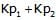= (x + y)2 → x + y =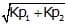→ total pressure = 2 (x + y) = 2=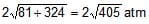(ii) mole ratio of B & D =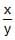=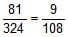=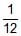(iii) mole fraction of B in mixture =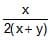=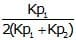Heterogeneous reactions: the vapor pressure of solid hydrates

Many common inorganic salts form solids which incorporate water molecules into their crystal structures.

These water molecules are usually held rather loosely and can escape as water vapor. Copper(II) sulfate, for example forms a pentahydrate in which four of the water molecules are coordinated to the Cu2 ion while the fifth is hydrogen-bonded to SO4--. This latter water is more tightly bound, so that the pentahydrate loses water in two stages on heating: CuSO4.5H2O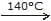CuSO4.H2O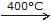CuSO4

These dehydration steps are carried out at the temperatures indicated above, but at any

temperature, some moisture can escape from a hydrate. For the complete dehydration of the

pentahydrate we can define an equilibrium constant CuSO4.5H2O(s) → CuSO4(s)  + 5 H2O(g) Kp = 1.14×10-10

The vapor pressure of the hydrate (for this reaction) is the partial pressure of water vapor at which the two solids can coexist indefinitely; its value is Kp1/5 atm. If a hydrate is exposed to air in which the partial pressure of water vapor is less than its vapor pressure, the reaction will proceed to the right and the hydrate will lose moisture. Vapor pressures always increase with temperature, so any of these compounds can be dehydrated by heating. Loss of water usually causes a breakdown in the structure of the crystal; this is commonly seen with sodium sulfate, whose vapor pressure is sufficiently large that it can exceed the partial pressure of water vapor in the air when the relative humidity is low. What one sees is that the well-formed crystals of the decahydrate undergo deterioration into a powdery form, a phenomenon known as efflorescence. When a solid is able to take up moisture from

the air, it is described as hygroscopic. A small number of anhydrous solids that have low vapor pressures not only take up atmospheric moisture on even the driest of days, but will become wet as water molecules are adsorbed onto their surfaces; this is most commonly observed with sodium hydroxide and calcium chloride. With these solids, the concentrated solution that results continues to draw in water from the air so that the entire crystal eventually dissolves into a puddle of its own making; solids exhibiting this behavior are said to be deliquescent.

 name formula vapor pressure, 25°C Torr 30°C sodium sulfate decahydrate Na2SO45H20 19.2 25.3 copper sulfate pentahydrate CuS04-5H20 7.8 12.5 calcium chloride monohydrate CaCl2H20 3.1 5.1 (water) H20 23.5 31.6

One of the first hydrates to be investigated in detail was calcium sulfate hemihydrate (CaSO4×1/2 H2O) which LeChâtelier showed to be what forms when when the form of CaSO4 known as plaster of Paris hardens; the elongated crystals of the hydrate bind themselves into a cement-like mass.

Ex. At what relative humidity will copper sulfate pentahydrate lose its waters of hydration when the air temperature is 30°C? What is Kp for this process at this temperature?

Sol. From the table, we see that the vapor pressure of the hydrate is 12.5 torr, which corresponds to a relative humidity of 12.5/31.6 = 0.40 or 40%. This is the humidity that will be maintained if the hydrate is placed in a closed container of dry air.

For this hydrate, Kp =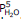, so the partial pressure of water vapor that will be in equilibrium with the hydrate and the dehydrated solid (remember that both solids must be present to have equilibrium!),expressed in atmospheres, will be (12.5/760)5= 1.20 x10-9.

Relationship Between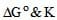Free energy, G, denotes the self intrinsic electrostatic potential energy of a system. This means that in any molecule if we calcualte the total electrostatic potential energy of all the charges due to all the other charges , we get what is called the free energy of the molecule. It tells about the stability of a molecule with respect to another molecule. Lesser the free energy of a molecule more stable it is.Every reaction proceeds with a decrease in free energy.The free energy change in a process is expressed by ΔG.If it is negative, it means that product have lesser G than reactants, so the reaction goes forward.If it is positive the reaction goes reverse and if it is zero the reaction is at equilibrium.

ΔG is the free energy change at any given concentration of reactants and products . If all the reactants and products are taken at a concentration of 1 mole per liter, the free energy change of the reaction is called ΔG( standard free energy change ).

One must understand that ΔGis not the free energy change at equilibrium . It is free energy change when all the reactants and products are at a concentration of 1 mole/L . ΔGo is related to K (equilibrium constant ) by the relation , ΔGo= -RT ln K.

K may either be kc or Kp.Accordingly we get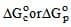. The units of DGdepends only or R.

T is always is Kelvin, and if R is in Joules, ΔGwill be in joules, and if R is calories than ΔGo will be in calories.

The document Industrial Applications of Le Chatelier's Principle & Haber Process - Notes | Study Chemistry Class 11 - NEET is a part of the NEET Course Chemistry Class 11.
All you need of NEET at this link: NEET

## Chemistry Class 11

204 videos|331 docs|229 tests

## Chemistry Class 11

204 videos|331 docs|229 tests

### How to Prepare for NEET

Read our guide to prepare for NEET which is created by Toppers & the best Teachers

Track your progress, build streaks, highlight & save important lessons and more!

,

,

,

,

,

,

,

,

,

,

,

,

,

,

,

,

,

,

,

,

,

;